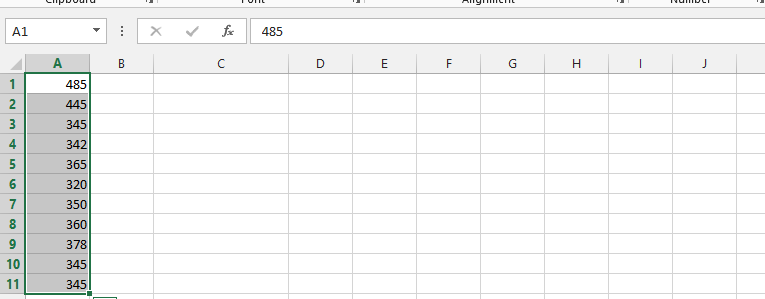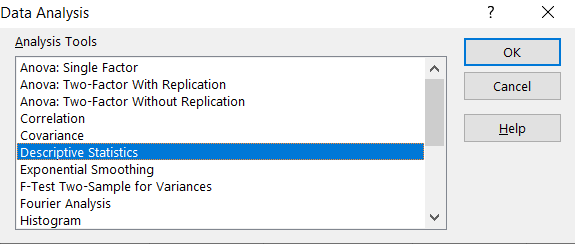### How to calculate Standard Deviation in Excel

The standard deviation is a mathematical term, and symbolizes the measurement of how spread out outlaid numbers are. We are going to make the standard deviation calculation.

### Example 1 How to calculate Standard Deviation using STDEV Excel function?

Let’s start from preparing your data. For this data we will see how to calculate Standard Deviation here.Click on a blank column and type in =STDEV(choose all columns with numbers in it ex. A1:A11), and then press enter.The Standard Deviation is calculated.Simple and quick. Luckily Excel has dedicated STDEV function for Standard Deviation calculations. There are also other Excel functions:

• STDEV.P – standard deviation of population
• STDEV.S – sample standard deviation
• STDEVA – sample standard deviation which does not ignore logical values
• STDEVPA – standard deviation of population which does not ignore logical values

### Example 2 Calculate Standard Deviation using Data Analysis ToolPak Add-In

First you need to make sure your Data Analysis Toolpak Add-In is installed. Here is the lesson how to install Data Analysis Toolpak Add-In.

Go to Ribbon to the Data tab. Click the Data Analysis button.New window appears. Choose Descriptive Statistics and click OK.Enter the proper parameters:

• Input Range – range of your data (\$A\$1:\$A\$11 in my example)
• Labels in first row – there are no labels in my data table
• Output Range – choose where you want to get your standard deviation calculated (I chose the same Sheet \$F\$1:\$G\$14)
• Summary statistics – make sure it is checkedAnd this is how to see my Statistics summary. Standard Deviation is calculated in row 7. The result is the same as in the Example 1 where we checked how to calculate Standard Deviation using STDEV Excel function.Excel really helps you with statistics. Hopefully now you can see that.

## Template

```Further reading: